Q-Chem 4.3 User’s Manual

# 6.9 Restricted active space spin-flip (RAS-SF) and configuration interaction (RAS-CI) methods

The restricted active space spin-flip (RAS-SF) is a special form of configuration interaction that is capable of describing the ground and low-lying excited states with moderate computational cost in a single-reference formulation [390, 391, 392, 393], including strongly correlated systems. The RAS-SF approach is essentially a much lower computational cost alternative to Complete Active Space SCF (CAS-SCF) methods. RAS-SF typically works by performing a full CI calculation within an active space that is defined by the half-occupied orbitals of a restricted open shell HF (ROHF) reference determinant. In this way the difficulties of state-specific orbital optimization in CAS-SCF are bypassed. Single excitations into (hole) and out of (particle) the active space provide state-specific relaxation instead. Unlike most CI-based methods, RAS-SF is size-consistent, as well as variational, and, the increase in computational cost with system size is modest for a fixed number of spin flips. Beware, however, for the increase in cost as a function of the number of spin-flips is exponential! RAS-SF has been shown to be capable of tackling multiple low-lying electronic states in polyradicals and reliably predicting ground state multiplicities [390, 394, 395, 396, 391, 392].

RAS-SF can also be viewed as one particular case of a more general RAS-CI family of methods. For instance, instead of defining the active space via spin-flipping as above, initial orbitals of other types can be read in, and electronic excitations calculated this way may be viewed as a RAS-EE-CI method (though size-consistency will generally be lost). Similar to EOM-CC approaches (see Section 6.7), other target RAS-CI wavefunctions can be constructed starting from any electronic configuration as the reference and using a general excitation-type operator. For instance, one can construct an ionizing variant that removes an arbitrary number of particles that is RAS-IP-CI. An electron attaching variant is RAS-EA-CI .

Q-Chem features two versions of RAS-CI code with different, complementary, functionalities. One code (invoked by specifying CORR=RASCI) has been written by David Casanova ; below we will refer to this code as RASCI1. The second implementation (invoked by specifying CORR=RASCI2) is primarily by Paul Zimmerman ; we will refer to it as RASCI2 below.

The RASCI1 code uses an integral-driven implementation (exact integrals) and spin-adaptation of the CI configurations which results in a smaller diagonalization dimension. The current Q-Chem implementation of RASCI1 only allows for the calculation of systems with an even number of electrons, with the multiplicity of each state being printed alongside the state energy. Shared memory parallel execution decreases compute time as all the underlying integrals routines are parallelized.

The RASCI2 code includes the ability to simulate closed and open shell systems (i.e., even and odd numbers of electrons), fast integral evaluation using the resolution of the identity (RI) approximation, shared memory parallel operation, and analysis of thevalues and natural orbitals. The natural orbitals are stored in the QCSCRATCH directory in a folder called “NOs” in Molden-readable format. Shared memory parallel is invoked as described in Section 2.7.1. A RASCI2 input requires the specification of an auxiliary basis set analogous to RI-MP2 computations (see Section 5.5.1). Otherwise, the active space as well as hole and particle excitations are specified in the same way as in RASCI1.

Note: Because RASCI2 uses the RI approximation, the total energies computed with the two codes will be slightly different; however, the energy differences between different states should closely match each other.

## 6.9.1 The Restricted Active Space (RAS) Scheme

In the RAS formalism, we divide the orbital space into three subspaces called RAS1, RAS2 and RAS3 (Fig. 6.2). The RAS-CI states are defined by the number of orbitals and the restrictions in each subspace.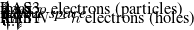Figure 6.2: Orbital subspaces in RAS-CI employing a ROHF triplet reference.

The single reference RAS-CI electronic wavefunctions are obtained by applying a spin-flipping or excitation operator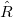on the reference determinant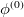.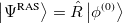(6.56)

Theoperator must obey the restrictions imposed in the subspaces RAS1, RAS2 and RAS3, and can be decomposed as: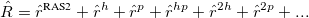(6.57)

where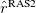contains all possible electronic promotions within the RAS2 space, that is, a reduced full CI, and the rest of the terms generate configurations with different number of holes (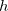superindex) in RAS1 and electrons in RAS3 (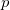superindex). The current implementation truncates this series up to the inclusion of hole and particle contributions, i.e. the first three terms on the right hand side of Eq. (6.57).

## 6.9.2 Job Control for the RASCI1 implementation

At present the RASCI1 and RASCI2 implementations employ different keywords (which will be reconciled in a future version). This subsection applies to RASCI1 (even electron systems, spin adapted algorithm using exact integrals).

## 6.9.4 Examples

Example 6.169  Input for a RAS-2SF-CI calculation of three states of the DDMX tetraradical using RASCI1. The active space (RAS2) contains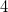electrons in thesingly occupied orbitals in the ROHF quintet reference. Natural orbital occupancies are requested.

$molecule 0 5 C 0.000000 0.000000 1.092150 C -1.222482 0.000000 0.303960 C -2.390248 0.000000 1.015958 H -2.344570 0.000000 2.095067 H -3.363161 0.000000 0.537932 C -1.215393 0.000000 -1.155471 H -2.150471 0.000000 -1.702536 C 0.000000 0.000000 -1.769131 C 1.215393 0.000000 -1.155471 H 2.150471 0.000000 -1.702536 C 1.222482 0.000000 0.303960 C 2.390248 0.000000 1.015958 H 2.344570 0.000000 2.095067 H 3.363161 0.000000 0.537932$end

$rem jobtype sp exchange hf correlation rasci basis 6-31g unrestricted false mem_total 4000 mem_static 100 ras_roots 3 ras_act 4 ras_elec 4 ras_occ 25 ras_spin_mult 0 ras_natorb true$end


Example 6.170  Input for a RAS-2IP-CI calculation of triplet states of Fmolecule using the dianion closed shell F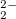as the reference determinant. RASCI1 code is used

$molecule -2 1 F F 1 rFF rFF = 1.4136$end

$rem jobtype sp exchange hf correlation rasci basis cc-pVTZ mem_total 4000 mem_static 100 ras_roots 2 ras_act 6 ras_elec 10 ras_occ 4 ras_spin_mult 3$end


Example 6.171  Input for a FCI/6-31G calculation of water molecule expanding the RAS2 space to the entire molecular orbital set. RASCI code is used.

$molecule 0 1 O 0.000 0.000 0.120 H -0.762 0.000 -0.479 H 0.762 0.000 -0.479$end

$rem jobtype sp exchange hf correlation rasci basis 6-31G mem_total 4000 mem_static 100 ras_roots 1 ras_act 13 ras_elec 10 ras_occ 0 ras_spin_mult 1 ras_do_hole false ras_do_part false$end


Example 6.172  Methylene single spin-flip calculation using RASCI2

$rem jobtype sp exchange HF correlation RASCI2 basis cc-pVDZ aux_basis rimp2-cc-pVDZ unrestricted false RAS_ACT_OCC 1 ! # alpha electrons RAS_ACT_VIR 1 ! # virtuals in active space RAS_ACT_DIFF 0 ! # set to 1 for odd # of e-s ras_n_roots 4 set_iter 25 ! number of iterations in RASCI$end

$molecule 0 3 C 0.0000000 0.0000000 0.0000000 H -0.8611113 0.0000000 0.6986839 H 0.8611113 0.0000000 0.6986839$end


Example 6.173  Two methylene separated by 10 ; double spin-flip calculation using RASCI2. Note that thevalues for this case will not be uniquely defined at the triply-degenerate ground state.

$rem exchange HF correlation RASCI2 basis cc-pVDZ aux_basis rimp2-cc-pVDZ RAS_ACT_OCC 2 ! # alpha electrons RAS_ACT_VIR 2 ! # virtuals in active space RAS_ACT_DIFF 0 ! # set to 1 for odd # of e-s unrestricted false jobtype sp ras_n_roots 8 set_iter 25$end

$molecule 0 5 C 0.0000000 0.0000000 0.0000000 H -0.8611113 0.0000000 0.6986839 H 0.8611113 0.0000000 0.6986839 C 0.0000000 10.0000000 0.0000000 H -0.8611113 10.0000000 0.6986839 H 0.8611113 10.0000000 0.6986839$end


Example 6.174  RASCI2 calculation of the nitrogen cation using double spin-flip.

$rem exchange HF correlation RASCI2 jobtype sp basis 6-31G* aux_basis rimp2-VDZ RAS_ACT_OCC 3 ! # alpha electrons RAS_ACT_VIR 3 ! # virtuals in active space RAS_ACT_DIFF 1 ! # for odd # e-s, cation unrestricted false n_frozen_core 2 n_frozen_virtual 2 ras_n_roots 8 set_iter 25$end

$molecule 1 6 N 0.0000000 0.0000000 0.0000000 N 0.0000000 0.0000000 4.5$end